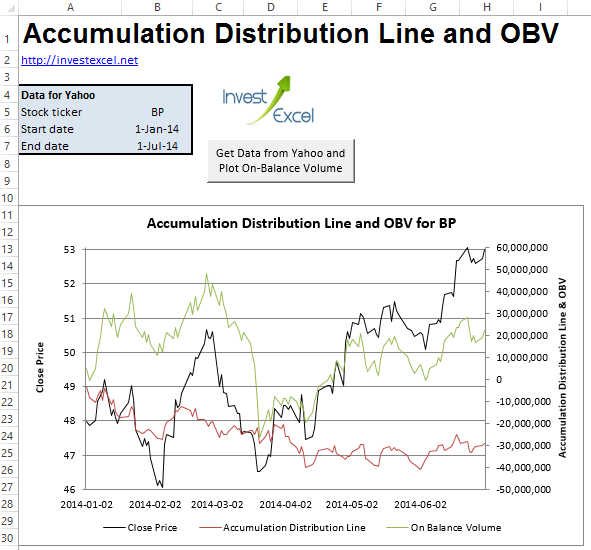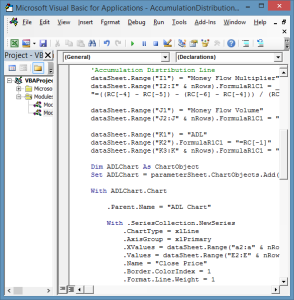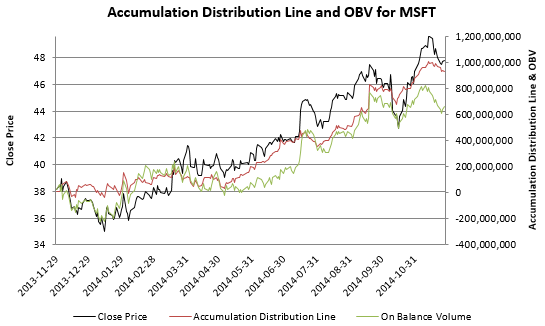# Accumulation Distribution Line

Detect hidden buying or selling pressure with accumulation distribution line

Developed by analyst Marc Chaikin, the accumulation-distribution line (ADL) measures the volume of money flowing into or out of a stock.

Technical traders use ADL as a proxy for underyling buying and selling pressure.

It’s commonly employed to confirm the predictions of other indicators (commonly RSI), and is a close-relation of on-balance volume.

Excel can help you calculate and plot ADL using a little VBA. You can download a web-connected VBA-powered spreadsheet from the link at the bottom of this post, but first let’s review the key formula and calculations.Step 1: For day n, calculate the Close Location Value (CLV), also known as the Money Flow Multiplier.Step 2: Then calculate the money flow volume.Step 3: Finally, calculate the accumulation-distribution line.CLV measures the distance of the close price from the high or low, and ranges between -1 and 1. If CLV is

• positive, the close is nearer to the high than the low
• negative, the close is nearer to the low than the high
• equal to 0, the close is midway between the high and the low

ADL is often used to confirm trends or predict reversals. For example, if prices track upwards but the ADL falls, a price reversal might be imminent. This is because falling ADL is associated with selling pressure.

This Excel spreadsheet employs some clever VBA to

• downloads stock data from Yahoo Finance
• programatically write the formulas to calculate ADL and OBV
• and generate charts plotting both against the close price

Here’s a snippet of the VBA – the part that programmatically writes the formulas that calculate ADL and OBV into a range of cells.

```'On Balance Volume dataSheet.Range("H1") = "OBV" dataSheet.Range("H2").FormulaR1C1 = "= RC[-2]" dataSheet.Range("H3:H" & nRows).FormulaR1C1 = _ "=IF(RC[-3] = R[-1]C[-3], R[-1]C, IF(RC[-3] > R[-1]C[-3], R[-1]C + RC[-2], R[-1]C - RC[-2] ))"   'Accumulation Distribution Line dataSheet.Range("I1") = "Money Flow Multiplier" dataSheet.Range("I2:I" & nRows).FormulaR1C1 = _ "=((RC[-4] - RC[-5]) - (RC[-6] - RC[-4])) / (RC[-6]-RC[-5]) "   dataSheet.Range("J1") = "Money Flow Volume" dataSheet.Range("J2:J" & nRows).FormulaR1C1 = "= RC[-1] * RC[-4]"   dataSheet.Range("K1") = "ADL" dataSheet.Range("K2").FormulaR1C1 = "=RC[-1]" dataSheet.Range("K3:K" & nRows).FormulaR1C1 = "= R[-1]C + RC[-1]"```

This is the ADL and OBV of Microsoft (MSFT) from 28th November 2013 to 28th November 2014 generated by this spreadsheet . The ADL and OBV rise in tandem with the price, confirming a strong trend.The code can be viewed, edited and modified. Please link to http://investexcel.net if you like this tool.

## 2 thoughts on “Accumulation Distribution Line”

1. Brian Menzies says:

Hi,Simar
Thank you for the many interesting sheets tou share on your site. Regarding the ADL & OBV chart, with stocks listed on the ASX the( red line ) ADL is vertical, I do not know if I can do anything to correct this or will the on balance and price give me enough information to give me the trend

Thank ypou
Brian Menzies

1. Brian

I checked out BHP.AX, and yes, the ADL line is horizontal (not vertical). This seems to be because the trading volume is sometimes zero – this messes up the ADL calculations (but not OBV).

I can catch this condition in the VBA and code around this. Wait for an updated sheet.

Samir